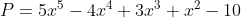# Test Prep Plan - Take a practice test

Take this practice test to check your existing knowledge of the course material. We'll review your answers and create a Test Prep Plan for you based on your results.
How Test Prep Plans work
1
2Based on your results, we'll create a customized Test Prep Plan just for you!
3Study smarter
Study more effectively: skip concepts you already know and focus on what you still need to learn.

# TASC Mathematics: Prep and Practice Final Exam

Free Practice Test Instructions:

Choose your answer to the question and click 'Continue' to see how you did. Then click 'Next Question' to answer the next question. When you have completed the free practice test, click 'View Results' to see your results. Good luck!

#### Question 3 3. A trucking company is offering a customer three different pricing options. Under which plan will the customer spend the least amount of money if her business requires a daily travel distance of 350 km?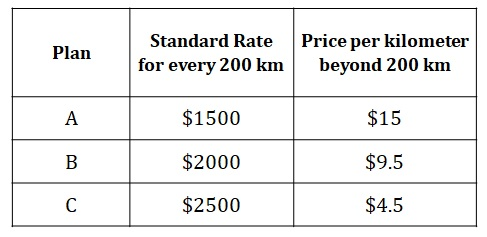#### Question 4 4. The table shows the proportional relationship between the number of hours, x, spent digging and the depth, y, of a hole in the ground. How many hours will it take to reach a depth of 49 meters?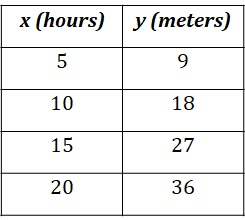#### Question 6 6. Given the values shown in the table above, what is the value of (f o g)(3)?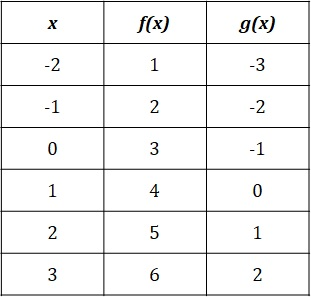#### Question 7 7. A boy kicked a ball from atop a building, throwing it in a direction that follows an inverted parabola. If the path of the ball can be modeled by the function, where y is the height in meters and x is the time in seconds, how long will it take for the ball to reach the ground?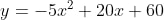#### Question 9 9. What is the equation of the function shown in the graph?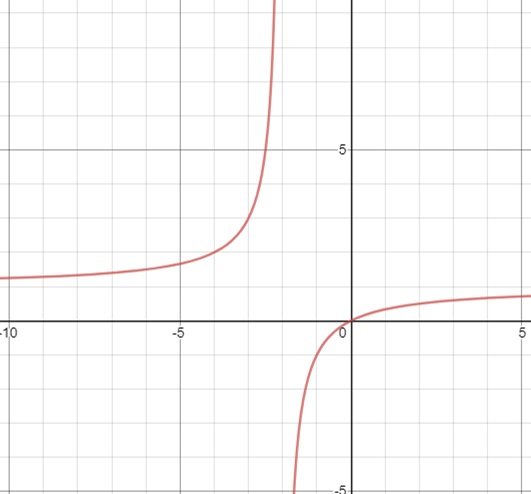#### Question 15 15. The population of a bacteria can be modeled by the function, where x is the time in hours. If the number of colonies can be modeled by the function N = x2 - x + 2, what function can be used to determine the number of bacteria per colony?